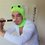# Need Help Solving these problems of Partial Differentiation

$$\large 1.$$ GIVEN: $$\large F(v^2-x^2,v^2-y^2,v^2-z^2)=0$$

where $\large v$ is a function of $x,y,z$

$\textbf{SHOW THAT:}$ $\large \frac{1}{x}\frac{\partial v}{\partial x}+\frac{1}{y}\frac{\partial v}{\partial y}+\frac{1}{z}\frac{\partial v}{\partial z}=\frac{1}{v}$

$\large 2.$ GIVEN: $V=f(X,Y,Z)$ is a homogeneous function in $x,y,z$ of degree $\boxed{n}$

where $X=\dfrac{\partial V}{\partial x}, Y=\dfrac{\partial V}{\partial y}, Z=\dfrac{\partial V}{\partial z}$

$\textbf{SHOW THAT:}$ $\large X\frac{\partial V}{\partial X}+Y\frac{\partial V}{\partial Y}+Z\frac{\partial V}{\partial Z}=\frac{n}{n-1}V$

Now for the second one, i understand that the Euler's theroem is necessary but i can't proceed in a definite direction from thereNote by Aritra Jana
4 years, 8 months ago

This discussion board is a place to discuss our Daily Challenges and the math and science related to those challenges. Explanations are more than just a solution — they should explain the steps and thinking strategies that you used to obtain the solution. Comments should further the discussion of math and science.

When posting on Brilliant:

• Use the emojis to react to an explanation, whether you're congratulating a job well done , or just really confused .
• Ask specific questions about the challenge or the steps in somebody's explanation. Well-posed questions can add a lot to the discussion, but posting "I don't understand!" doesn't help anyone.
• Try to contribute something new to the discussion, whether it is an extension, generalization or other idea related to the challenge.

MarkdownAppears as
*italics* or _italics_ italics
**bold** or __bold__ bold
- bulleted- list
• bulleted
• list
1. numbered2. list
1. numbered
2. list
Note: you must add a full line of space before and after lists for them to show up correctly
paragraph 1paragraph 2

paragraph 1

paragraph 2

[example link](https://brilliant.org)example link
> This is a quote
This is a quote
    # I indented these lines
# 4 spaces, and now they show
# up as a code block.

print "hello world"
# I indented these lines
# 4 spaces, and now they show
# up as a code block.

print "hello world"
MathAppears as
Remember to wrap math in $$ ... $$ or $ ... $ to ensure proper formatting.
2 \times 3 $2 \times 3$
2^{34} $2^{34}$
a_{i-1} $a_{i-1}$
\frac{2}{3} $\frac{2}{3}$
\sqrt{2} $\sqrt{2}$
\sum_{i=1}^3 $\sum_{i=1}^3$
\sin \theta $\sin \theta$
\boxed{123} $\boxed{123}$

Sort by:

- 4 years, 8 months ago

@Aritra Jana sorry but I don't know much about partial derivatives.

- 4 years, 8 months ago

oh sorry, my bad. although , could you help me by tagging some who you think have a certain degree of knowledge in this field?

- 4 years, 8 months ago

- 4 years, 8 months ago

What have you done? What have you tried?

Staff - 4 years, 8 months ago

@Aritra Jana @Calvin Lin i am opening brilliant after 3 years. i think i have 2 solutions to problem 1 but i am not being able to post a picture.plz help

- 4 years, 4 months ago

Approaches

1. If you email it to me, I can upload it for you.
2. If you upload it to an image site like Imgur, you can then use the markedown syntax of ![](URL) to display images.

Staff - 4 years, 4 months ago